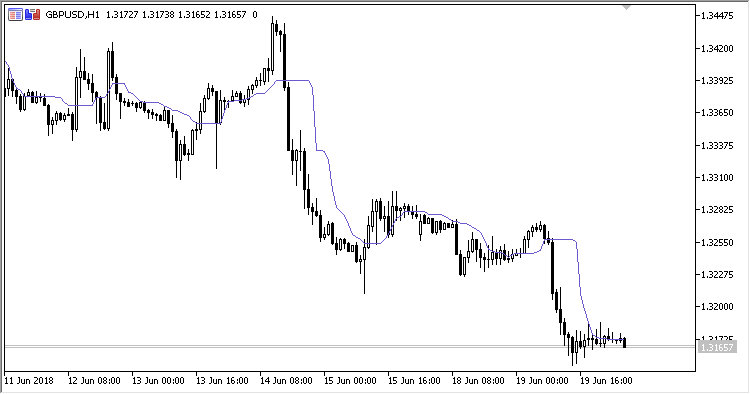# RMI – indicator for MetaTrader 5

• A+
category：MT5 INDICATORS

Indicator RMI (Running Median Indicator) is a moving average with the "Running Median" filtering algorithm.

It has two configurable parameters:

• Period - calculation period;
• Applied price - price used for calculations.

Calculations:

`RMI = VAR(Index)`

where:

• VAR - the values of Applied price in ascending order within Period.
`Index = floor(Length/2.0+0.5)`

If round(Period/2) * 2 = Period:

`Length = Period + 1`

Otherwise:

`Length = Period`